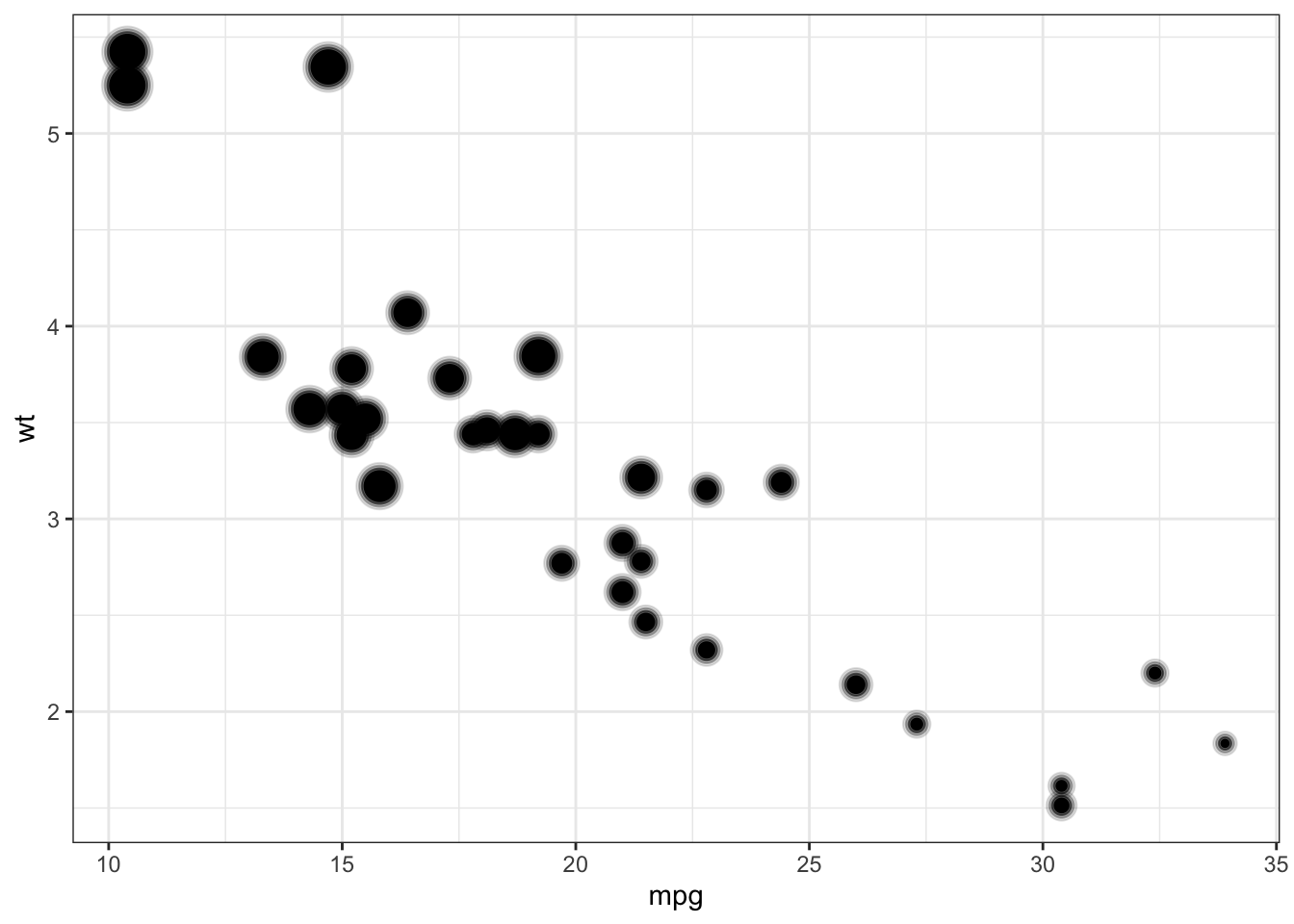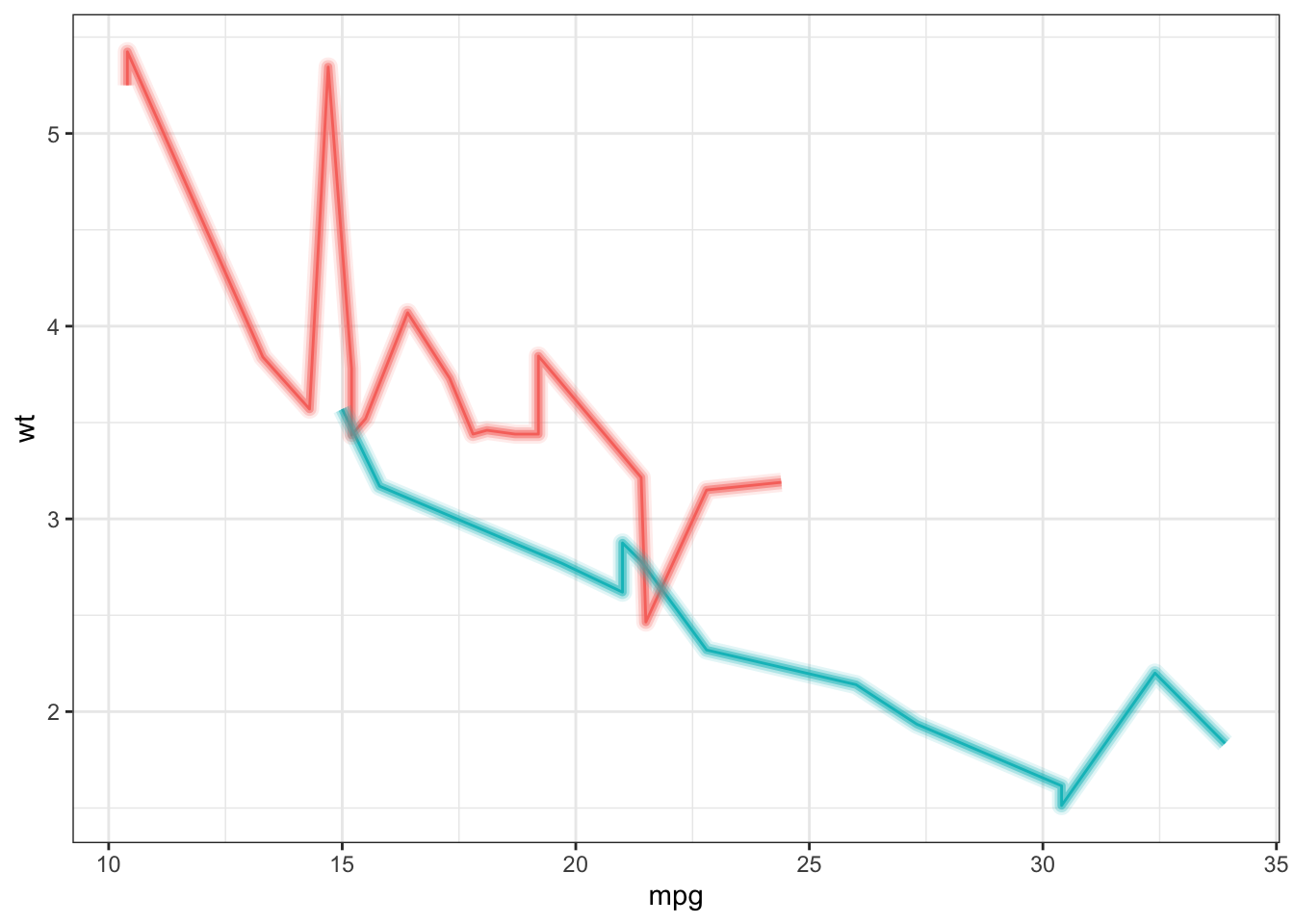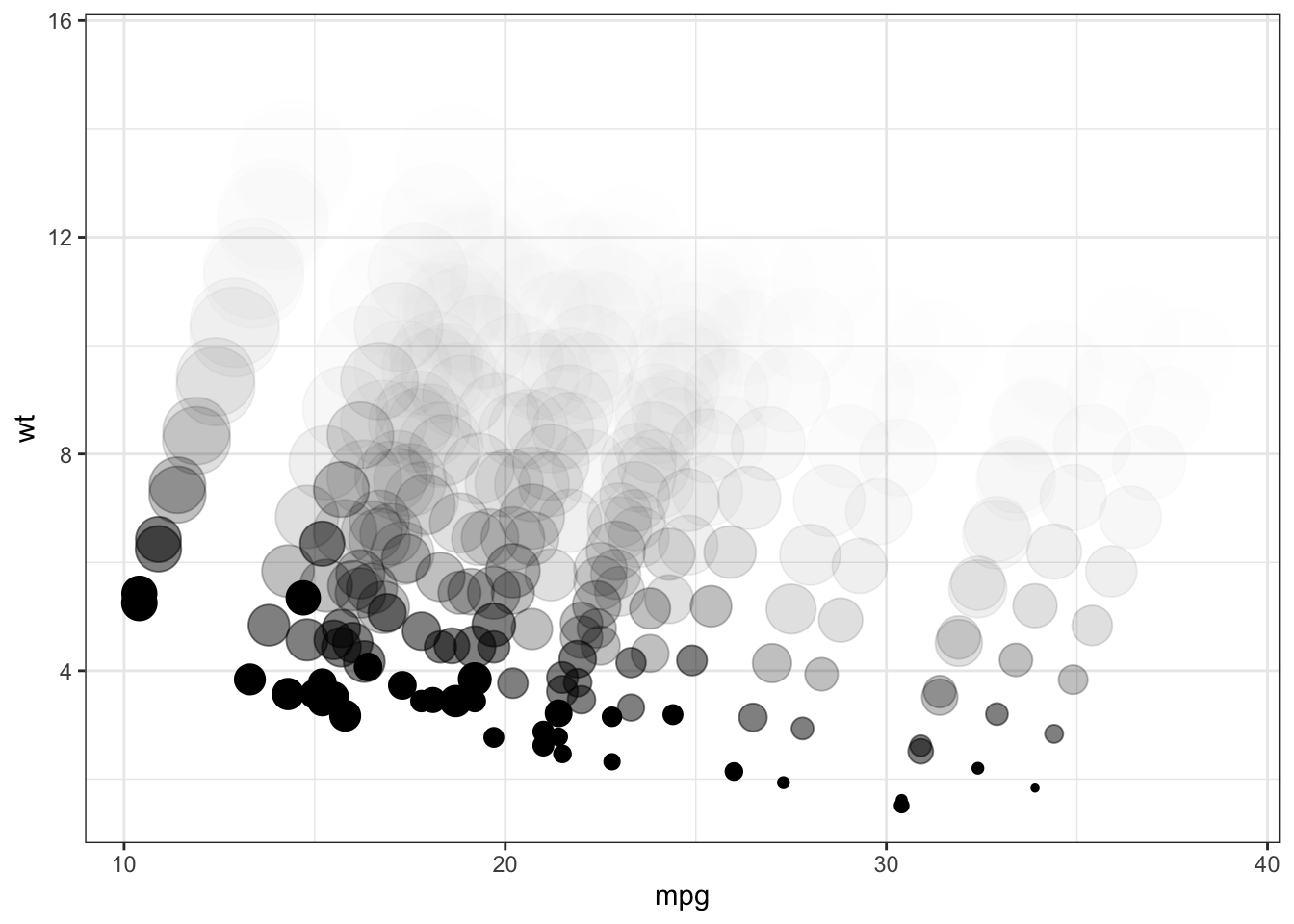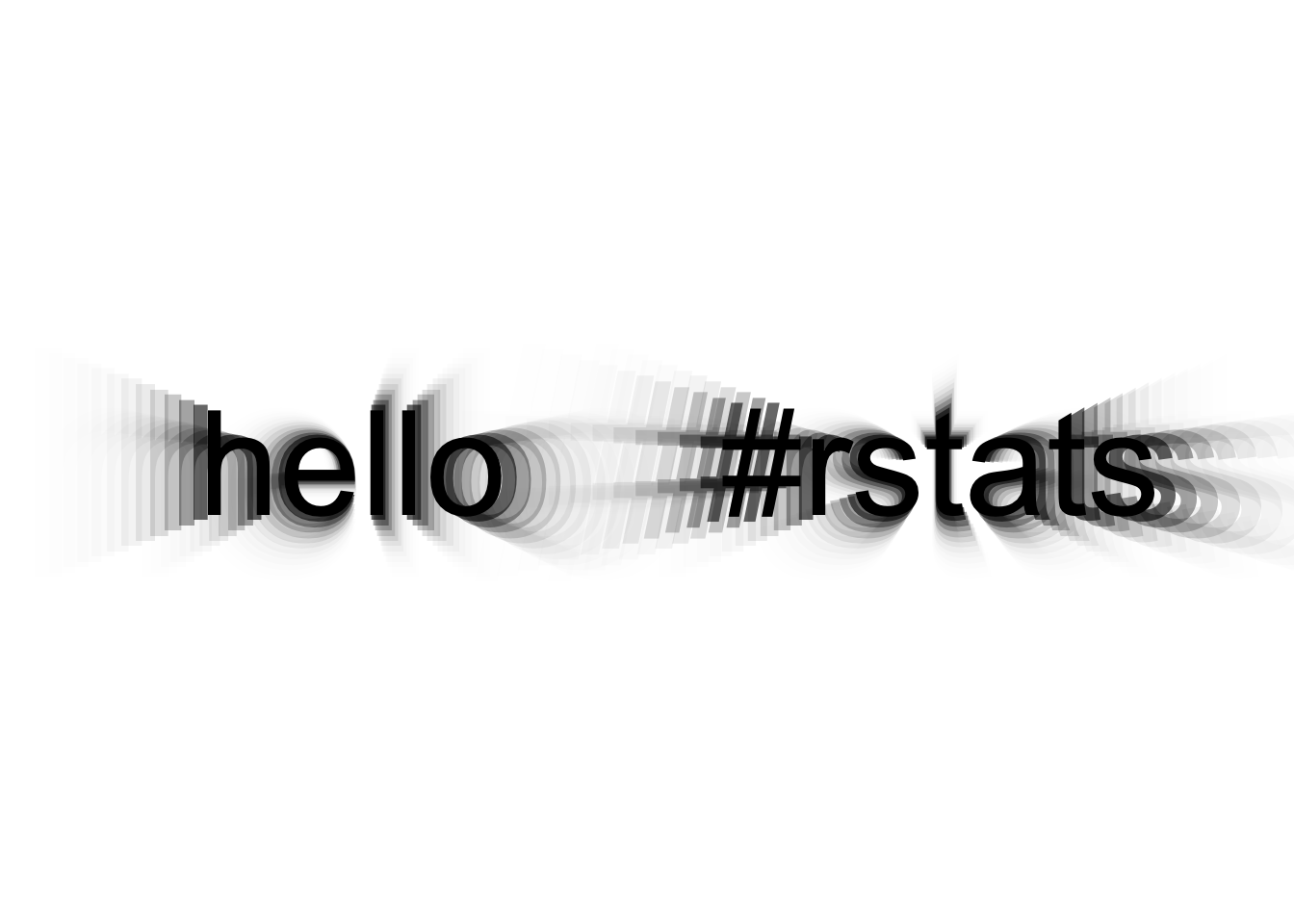# ggecho`ggecho` is a `ggplot2` Stat which echoes the plotting data.

This can be used to create a blur effect for `geom_point()`, `geom_line()` and `geom_text()` (and probably some other geoms where the `size` parameter directly determines the area of the displayed element).

This ggplot2 stat works by duplicating/echoing the original data passed to ggplot, and adjusting each data echo by:

• size (`size_increment`),
• alpha (`alpha_factor`), and
• position (`x_offset` & `y_offset`)

This package wouldn’t be possible without:

## Installation

You can install thedevelopment version from GitHub with:

``````# install.packages("remotes")
remotes::install_github("coolbutuseless/ggecho")``````

## Simple blurred points and lines

This is a basic example which shows you how to solve a common problem:

``````library(ggecho)

ggplot(mtcars) +
geom_point(aes(mpg, wt, size = disp), stat = "echo") +
theme_bw() +
theme(legend.position = 'none')````````````ggplot(mtcars) +
geom_line(aes(mpg, wt, colour = as.factor(am)), stat = "echo") +
theme_bw() +
theme(legend.position = 'none')````````````ggplot(mtcars) +
stat_echo(aes(mpg, wt, size = disp), geom = "point", size_increment = 2, alpha_factor = 0.5,
x_offset = 0.5, y_offset = 1, n = 10) +
theme_bw() +
theme(legend.position = 'none')``````## Blurred text

``````text_df <- data.frame(x = c(0.3, 0.7), y = c(0.25, 0.25), label = c('hello', '#rstats'))

ggplot(text_df) +
geom_text(aes(x, y, label = label), size = 20, stat = 'echo',
size_increment = 2, y_offset = 0.0, n = 20, alpha_factor = 0.6) +
theme_void() +
xlim(0.1, 0.9) + ylim(0.1, 0.4)``````